# Prism - Definition with Examples

The Complete K-5 Math Learning Program Built for Your Child

• 30 Million Kids

Loved by kids and parent worldwide

• 50,000 Schools

Trusted by teachers across schools

• Comprehensive Curriculum

Aligned to Common Core

## Prism Games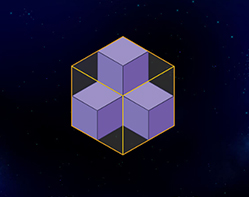Volume of Solids

Find the volume of solids by multiplying the area of the base by the height of the solid. Remember, that volume like area can be added.

Covers Common Core Curriculum 5.MD.5.c

## What is Prism

• A prism is a 3-dimensional shape with two identical shapes facing each other. These identical shapes are called “bases”.

• The bases can be a triangle, square, rectangle or any other polygon.

• Other faces of a prism are parallelograms or rectangles.

## Cross Section of Prisms

The cross section of a geometric shape or an object is the shape obtained by cutting it straight. It is also referred to as the intersection of a plane with the three-dimensional object. The cross section of a prism parallel to the base of the prism is same as its base.

• Triangular Prism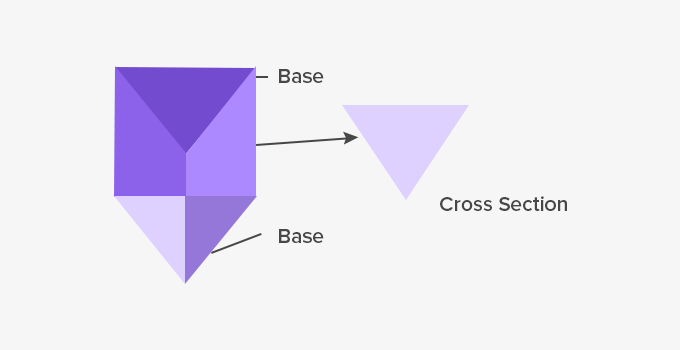• Cube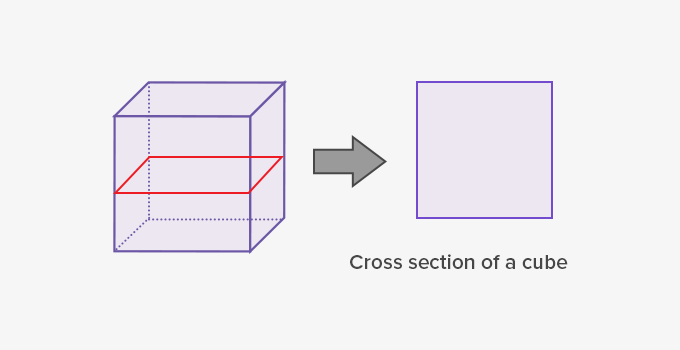## Regular and Irregular Prism

The base of a prism can be a regular or irregular polygon. Based on the shape of the base, prisms are regular or irregular prisms.

• Regular Prism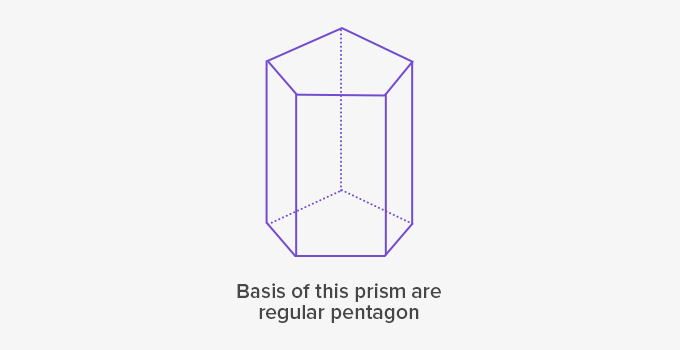• Irregular Prism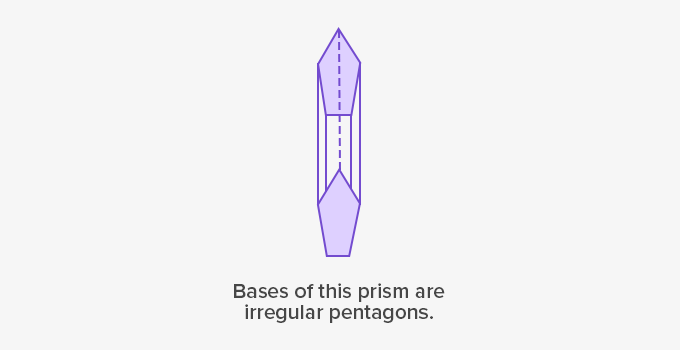## Surface Area and Volume of a Prism

The surface area of a prism is the sum of the area of all its faces.

Volume of a prism is the amount of space inside the prism.

Let us see how to find the surface area and volume of a triangular prism.

• Surface Area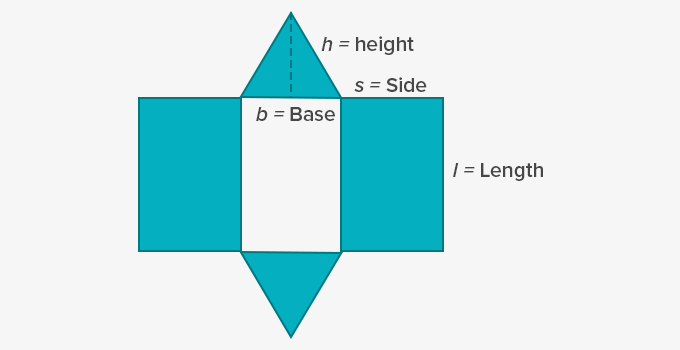Surface Area = Area of base triangles + Area of side parallelograms

= 2 × ( 1 2  x b x h) + 2 × (l x s) + (l x b)

= bh + 2ls + lb

• Volume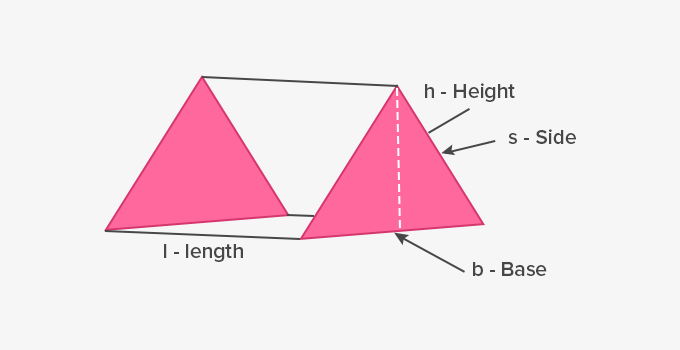Volume = Area of base triangle × length

= ( 1 2 b x h) × l

= 1 2 bhl

Example: Calculate the surface area and volume of the following prism.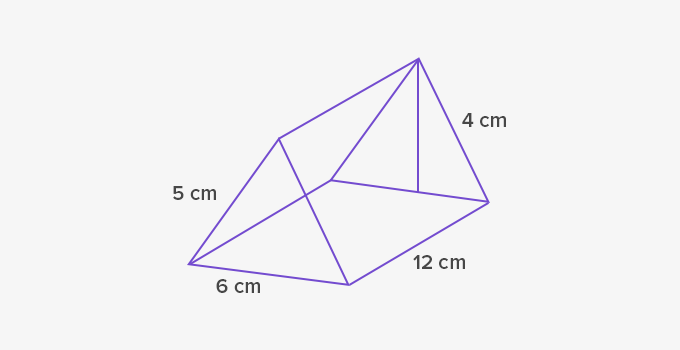Length (l) = 12 cm, Height (h) = 4 cm, Base (b) = 6 cm, Side (s) = 5 cm

 Surface area = bh+2ls+lb = 6 × 4 + 2 × 12 × 5 + 12 × 6  = 24 + 120 + 72  = 216 cm2 Volume = 1 2 bhl = 1 2 × 6 × 4 × 12 = 144 cm3

## Right Prism and Oblique Prism

When the two bases of a prism are perfectly aligned and its faces are rectangles (perpendicular to the bases) it is a right prism, else it is an oblique. They are characterized as follows:

• Right Prism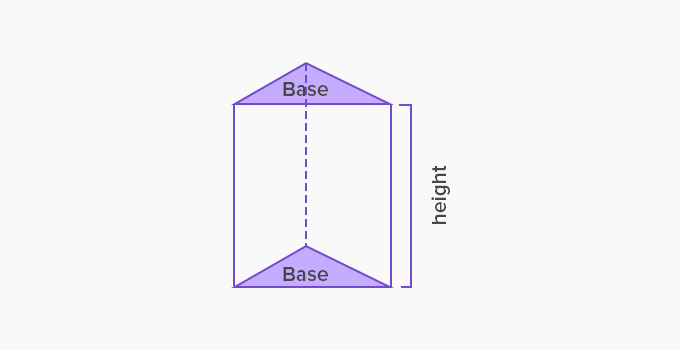• Oblique Prism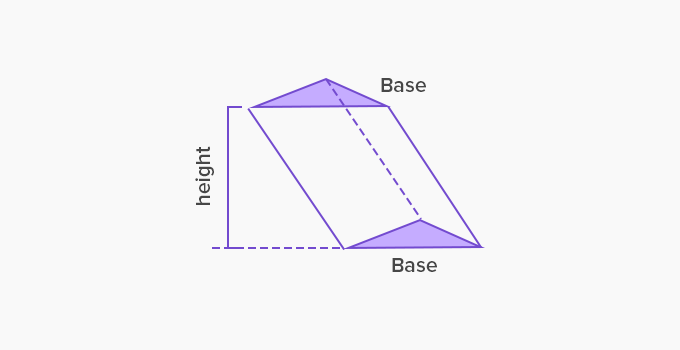Right Prism Oblique Prism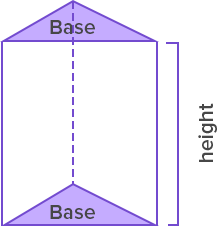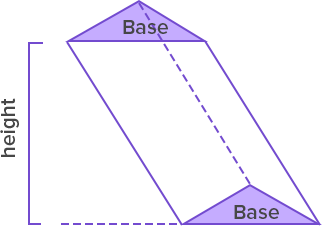Height The height is a lateral edge. Height is an altitude outside the prism. Side faces Side faces are rectangles. Sides faces are parallelograms. Surface Area bh+2ls+lb bh+2ls+lb Volume 1 2 bhl 1 2 bhl

 Fun Facts The prisms are polyhedrons or objects with multiple flat faces. A prism can not have any side which is curved thus objects like cylinder, cone or sphere are not prisms.

Won Numerous Awards & Honors